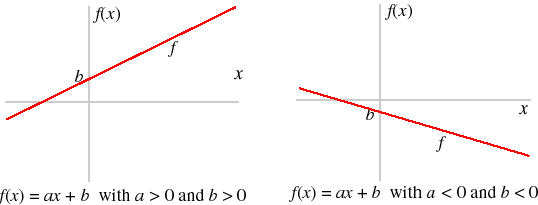# FUNCTIONS

FUNCTIONS. WHAT THEY ARE AND WHY THEY MATTER.

Functions are a crucial concept in mathematics. You get them in algebra, in geometry, in trigonometry. You hardly ever get them at parties, but that would depend on the nature of the party, I would guess. Today, I want to explore some of the properties of a function.

A function consists of a correspondence between a first and second set. Each element in the first set corresponds to exactly one element in the second set.

Take the following:

 A 1 B 2 C 3 D 4 E 5

This table denotes a function. For every item in the first column, there is a corresponding item in the second.

The first set is called the domain, the second, the range.

The domain is also the x-axis of a set of Cartesian Coordinates; the range is the y-axis.  This means that you can make a graph of a function!These are graphical depictions of functions.

Now, suppose you were to draw a vertical line through one of the lines already on the chart. It would be a function. As the line represents a point on the x-axis and goes through exactly one point on the y-axis. This is called the Vertical Line Test of functions.

The above graph shows the use of functions. If we had two sets of data, we could see correlations.

Say the x-axis represented increased gun violence and the y-axis gun ownership. The first would show a direct correlation between gun violence and gun ownership; the second an inverse correlation.Notice the vertical lines. The first defines a function, the second and third do not.This graph isn’t a purely functional graph. The y-axis is a bit crooked. But it would show a one-on-one correlation between the x-axis and the y-axis. (It also shows that as spending on education goes up, absolutely nothing happens to students’ skills, but you already knew that.)

And here’s a graph that is called a “best fit” graph, as you can’t draw a straight line.However, if you did draw a straight line, which is possible on the incident line without too much distortion, you would have a function. Mass shootings were 25 a year in 1980 and about 23 in 2010.

As should be clear by now, functions can be expressed by linear equations.  But instead of:

Y =2x+4

F(x)=2x +4.

We’ll continue this discussion in a future blog.

Dr. Fred Young

http://www.agapequalitytutorial.com